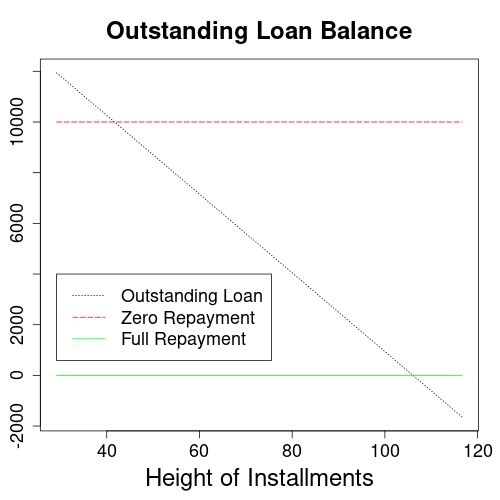Home » Interest Rate Calculators » Installment Loan Calculators » Outstanding Loan Amount Calculator for Installment Loans
Height of Installment Calculator for Installment Loans
Height of installment calculator for installment loan contracts calculates installments from the paid out loan and underlying interest rate.
Underlying Interest Rate Calculator for Installment Loans
Calculates the underlying interest rate of an installment loan from the start and end of term loan balances.

# Outstanding Loan Amount Calculator for Installment Loans

This financial calculator determines the outstanding loan amount at the end of the term of an installment loan. It is applicable to loan types with fixed interest and constant installments, such as mortgages, car loans and consumer credits. Usage instructions and explanations on the economics of installment loans follow after the calculation form.

 monthsyears

## Usage Instructions for the Outstanding Loan Amount Calculator

Please fill the calculator form with the amount of the loan paid out to you, the height of regular installments, the loan interest rate, and the term of the loan in years. All fields above the calculate button are input values, which you can change as needed. From these inputs the form will calculate the outstanding loan amount at the end of the contract.

The term of the loan is same as the contract duration, or the number of years you will have to pay off the loan.

Per default, the form sets twelve annual installments equivalent to monthly payments in its number of installments input field. Since mortgages or car loans usually require monthly payback, most users will be fine with this setting. However, you may override this to four (quarterly payments), two (semi-annual payments) or one (annual payments).

In addition to the end of term loan balance, the calculator outputs intermediate balances in the details section. Since this list can grow quite long, you may choose to hide it clicking the “hide -” label in its upper right corner.

## End of Term Outstanding Loan Dynamics

This section explains how the height of installments affects the end of term outstanding loan balance. It focuses on installments because this is where customers usually have the most choice in loan negotiations. With all other parameters fixed, there are two key levels of installment height: a lower one where the loan balance remains constant, and a higher one where the loan clears off completely.End of term outstanding loan balance for a \$10,000 loan with 10 year term and 5% interest.

The above graph shows how the loan balance remaining at the end of the term depends on the height of regular installments. The green line marks full repayment at the end of the term or contract. At the red line, installments merely cover interest and do not repay the loan. Because the total value of all installments is proportional to the height of installments, the outstanding balance drops in a straight line. It’s possible to repay the loan more than fully, in which case the remaining balance turns negative. Likewise, the remaining balance can grow if installments don’t even cover the interest.

## Mathematical Formula

The end of term loan balance calculator uses the following formula:

L1 = L0 * (1 + r/m)m*t - C0 * m/r * ((1 + r/m)m*t - 1)

L1: end of term loan balance
L0: initial loan balance
C0: height of installments
r: interest rate
t: duration of loan contract in years
m: number of installments per year

## References

Installment Loans: Wikipedia.org

## Similar Computations

Published: November 17, 2021
Updated: May 6, 2023

Financial Algebra
Financial Algebra